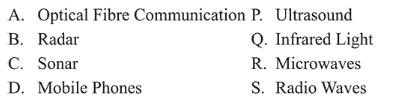# Given below in the left column are different modesQuestion:

Given below in the left column are different modes of communication using the kinds of waves given in the right column.From the options given below, find the most appropriate match between entries in the left and the right column.

1. (1) $A-Q, B-S, C-R, D-P$

2. (2) $0.4$ and $10 \mathrm{kHz}$

3. (3) $0.3$ and $9 \mathrm{kHz}$

4. (4) $0.5$ and $9 \mathrm{kHz}$

Correct Option: , 3

Solution:

(3) Optical Fibre Communication - Infrared Light